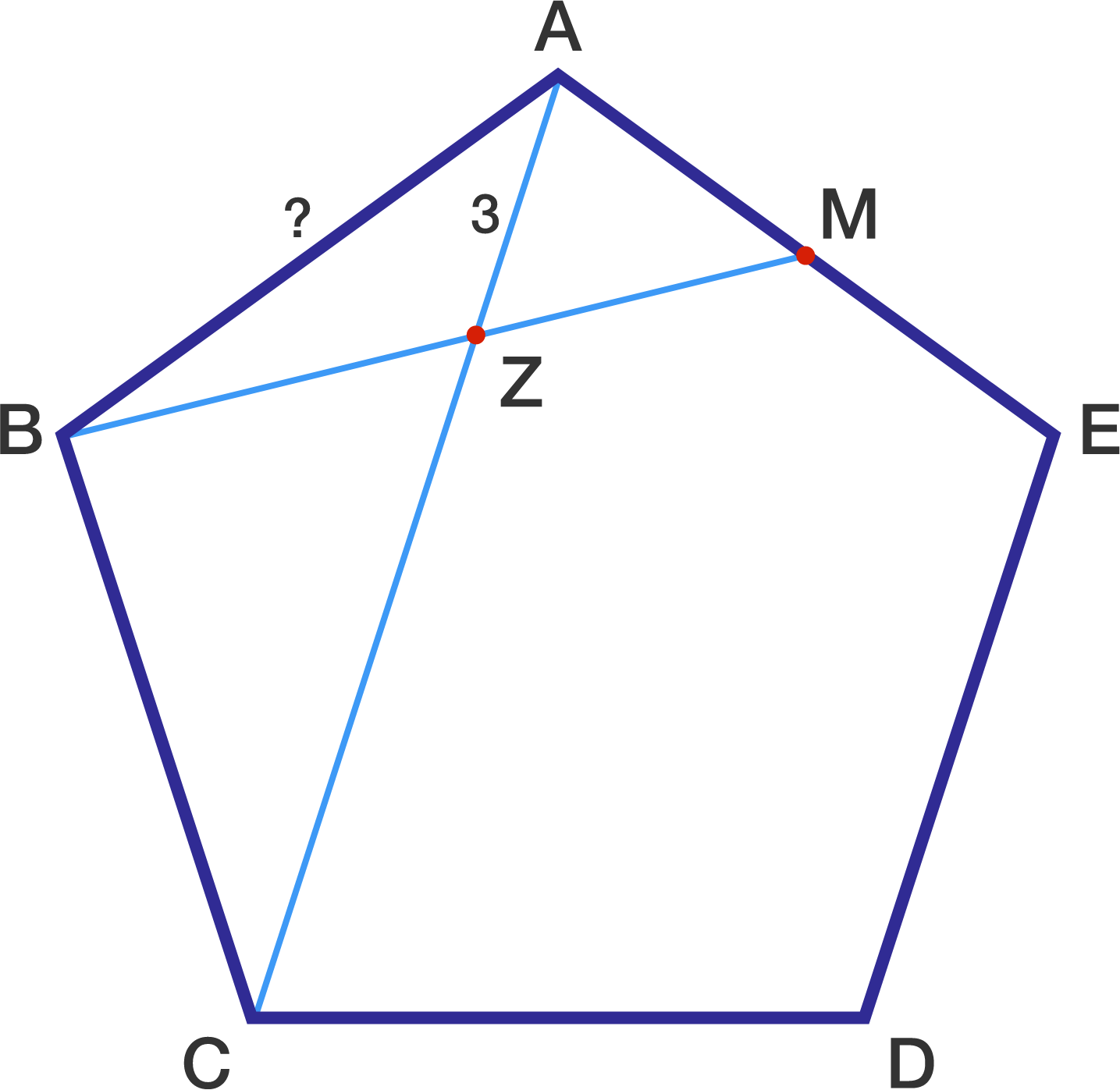# Pentagon Problem

Geometry Level 2In regular pentagon $ABCDE,$ point $M$ is the midpoint of side $AE.$

If line segments $AC$ and $BM$ intersect at point $Z$ and $ZA=3,$ what is the length of $AB?$

×